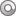# Cena代码评测软件系列之二：评测Java代码

```#include <cstring>
#include <cstdlib>
int main(int argc,char** argv){
//bc.exe %s
if(argc!=2)return 0;
std::string temp(argv,strlen(argv));
std::string cmd=temp+".exe <in.txt >out.txt";
system(cmd.c_str());
return 0;
/*
//并不需要自己写编译工具，g++即可
//ac.exe %s [path/to/g++]
if(argc!=2 && argc!=3)return 0;
string g="g++";
if(argc==3){
g=argv;
}
string cmd=g;
string temp(argv,strlen(argv));
cout<<temp<<endl;
cmd = cmd +" "+temp+".cpp -o "+temp+".exe";
cout<<cmd<<endl;
system(cmd.c_str());
return 0;
*/
}
```

```#include <fstream>
#include <iostream>
#include <cstdlib>
#include <cstring>
using namespace std;
int main(int argc,char** argv){
//ajavac.exe %s [path/to/javac]
if(argc!=2 && argc!=3){cout<<"Usage: path/to/ajavac.exe %s [path/to/javac]\n";return 0;}
string cmd="javac";
if(argc==3){
cmd=argv;
//可能有空格,所以要加引号：
//"D:\Program Files\Java\jdk1.8.0_05\bin\javac.exe"
cmd="\""+cmd+"\"";
}
string filename(argv,strlen(argv));
ifstream fin;
filename+=".java";
fin.open(filename.c_str(),ios_base::in);
char temp;
string rightname;
while(fin.get(temp) && temp!='\n'){
if(temp=='/')continue;
rightname+=temp;
}
cmd +=" "+rightname;
//复制
system(("copy "+filename+" "+rightname).c_str());
//编译
system(cmd.c_str());
return 0;
}
```

```#include <iostream>
#include <fstream>
#include <cstring>
#include <cstdlib>
using namespace std;
int main(int argc,char** argv){
//bjavac.exe %s [path/to/java.exe]
if(argc!=2 && argc!=3){cout<<"Usage: path/to/bjava %s\n";return 0;}
string cmd="java";
if(argc==3){
cmd=argv;
//可能有空格,所以要加引号：
//"D:\Program Files\Java\jdk1.8.0_05\bin\java.exe"
cmd="\""+cmd+"\"";
}
string filename(argv,strlen(argv));
filename += ".java";
ifstream fin(filename.c_str(),ios_base::in);
char temp;
string classname;
while(fin.get(temp) && temp!='.'){//A.java
if(temp=='/')continue;
classname+=temp;
}
cmd += " "+classname+" <in.txt >out.txt";
system(cmd.c_str());
return 0;
}
```

## “Cena代码评测软件系列之二：评测Java代码”上的3条回复[…] 上篇文章讲到约定源文件注释格式来提取正确文件名从而使用Cena评测Java代码，这篇文章分享使用php解析Cena生成的xml评测结果文件的方法从而使得选手能够在一轮评测后查看自己的成绩。 […]